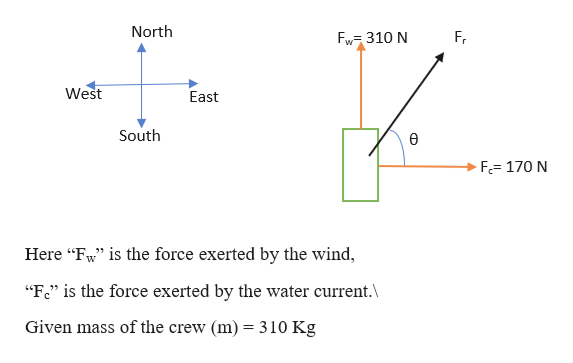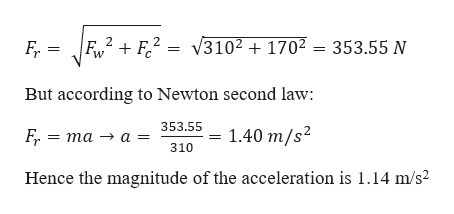# The force exerted by the wind on the sails of a sailboat is 310 N north. The water exerts a force of 170 N east. If the boat (including its crew) has a mass of 310 kg, what are the magnitude and direction of its acceleration?

Question
1 views

The force exerted by the wind on the sails of a sailboat is 310 N north. The water exerts a force of 170 N east. If the boat (including its crew) has a mass of 310 kg, what are the magnitude and direction of its acceleration?

check_circle

Step 1

Given situation can be picturized as follows:help_outlineImage TranscriptioncloseNorth Fw 310 N Fr West East South F= 170 N Here "Fw" is the force exerted by the wind, "Fe" is the force exerted by the water current.\ Given mass of the crew (m) = 310 Kg fullscreen
Step 2

Now the resultant force (Fr) c...help_outlineImage TranscriptioncloseFv2 F2 = V3102 1702 = 353.55 N Fr But according to Newton second law: 353.55 1.40 m/s2 F, 3D та — 310 Hence the magnitude of the acceleration is 1.14 m/s2 fullscreen

### Want to see the full answer?

See Solution

#### Want to see this answer and more?

Solutions are written by subject experts who are available 24/7. Questions are typically answered within 1 hour.*

See Solution
*Response times may vary by subject and question.
Tagged in

### Vectors and Scalars